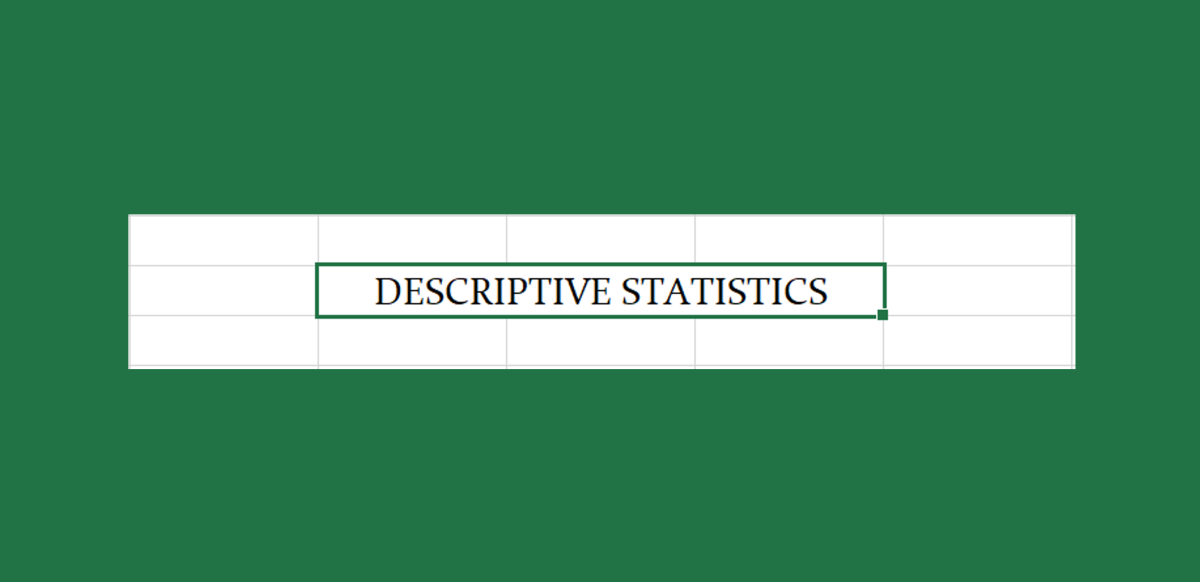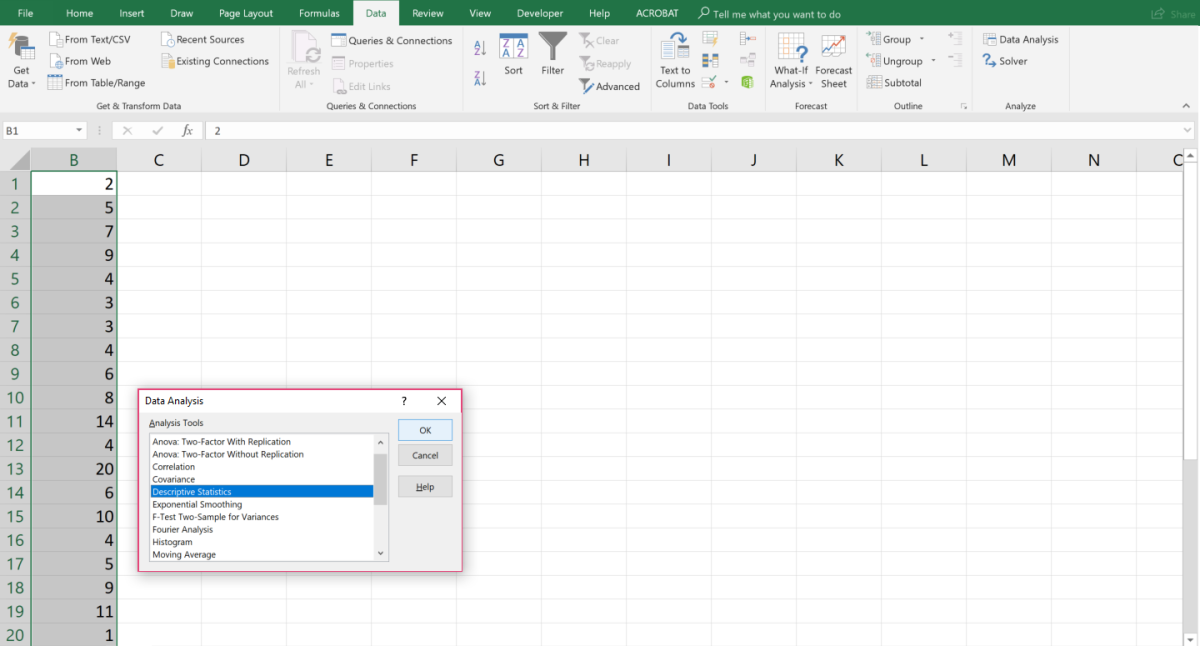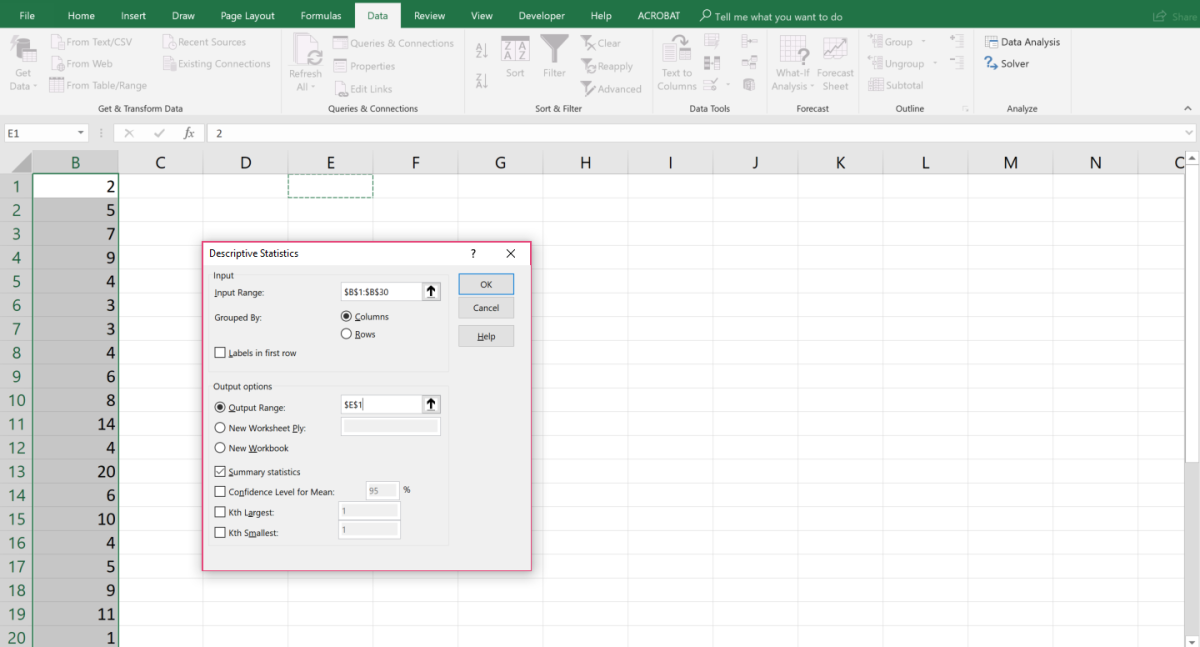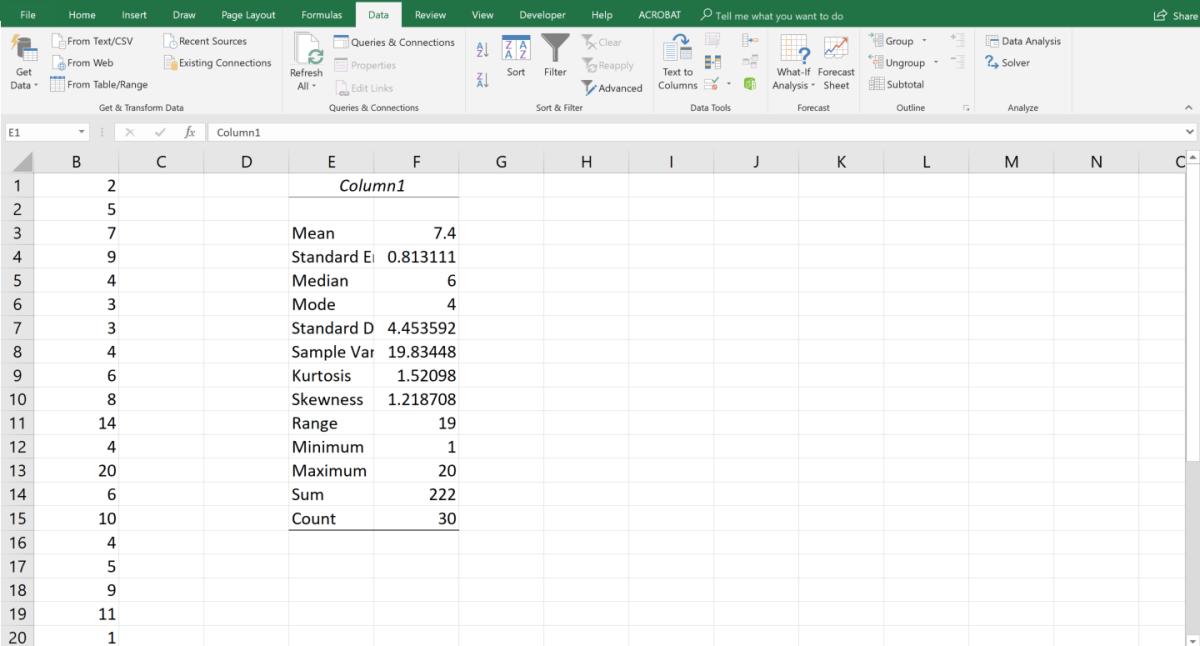# How to Use Microsoft Excel’s Data Analysis ToolPak for Descriptive Statistics

Joshua is a graduate student at the USF. He has interests in business technology, analytics, finance, and lean six sigma.Descriptive statistics describe the features of data by providing summaries about the sample or population being studied. With graphic analysis, descriptive statistics shape the basis of most quantitative analyses of data.Created by Joshua Crowder

Excel makes calculating statistics much easier today than ever before. It literally takes a few keystrokes and clicks to get just about any type of statistical measurement or graph from a data set. Excel is preloaded with statistical functions that can help you find the mean, median, mode, variance, and many more statistical measurements.
Aside from Excel's functions, the program also allows users the option to install a Data Analysis ToolPak Add-in that is used to perform many types of calculations at once. This tutorial shows an excel user how to use the Data Analysis tool to find descriptive statistics and explains the results.

## Activate the Data Analysis Data ToolPak

If you have never used the Data Analysis ToolPak, it is probably inactive on your Excel program. You can check to see if you have it by first clicking on the data tab. Next, look for the analysis group on the far-right side of your screen. If the data analysis option does not exist use the following steps to activate this add-in.

1. Click on the File tab, followed by clicking on options. Next, click on “Add-Ins.”
2. Next, click on the “Go” button to the manage add-ins section.
3. Lastly, check the “Analysis Pak” box and click “OK.”

You should now be ready to use the Data Analysis ToolPak from the data tab in the analysis group.

## Data Analysis Example

If following along with this example with an excel worksheet type this data set into Excel vertically in individual cells.

2, 5, 7, 9, 4, 3, 3, 4, 6, 8, 14, 4, 20, 6, 10, 4, 5, 9, 11, 1, 6, 9, 4, 5, 13, 18, 7, 6, 9, 10

Click on “Data Analysis” in the data tab and then click on Descriptive Statistics in the dialog box. Click the OK button.With the data analysis tool pak you can provide data and parameters for each analysis, and the data tool uses the right statistical macros in Excel to calculate the results in a table as output.Created by Joshua Crowder

Next, the range of the data needs to be typed in the input range section of the dialog box. Choose the output range option and choose a cell for the output to display by typing that cell location in the blank field. Lastly, click in the Summary statistics checkbox and click OK to display the results.Using the Descriptive Statistics analysis tool, a report can be generated that displays univariate statistics for data in the input range. This data provides information concerning the central tendency and variability of the data used.Created by Joshua Crowder

## The Results

The results print in two columns. The first column represents the descriptive statistic and the second column shows the results for those statistics. In the following sections, I will describe what these descriptive statistics represent.The Descriptive Statistics tool displays 13 statistical results so you can make a quick analysis.Created by Joshua Crowder

## Mean

The mean, which is a measure of central tendency, was calculated by taking the average of the whole data set. The sum of the data set is 222 and when divided by 30 gives a mean of 222/30 = 7.4. The mean can be written as the expression: ∑xi /n, where n is the number of values and ∑xi is the sum of data values.

Scroll to Continue

## Standard Error

The standard error is defined as the standard deviation divided by the square root of the sample size. In the above example, the standard error is 4.45/sqrt(30) = 0.813.

## Median

The median is the middle value and another measure of central tendency. To find the median or middle value of the data set, you need to arrange the whole data set in ascending or descending order. The median value is directly in the middle when there is an odd number of values in the data set. When there is an even number of values, there will be two middle values that need to be averaged to find the median.

## Mode

Another measure of central tendency is the mode. This is the value that appears more frequently than any other value. In the example above, 4 appears more than any other number. There may be a case where there is more than one mode. This occurs when two numbers appear most frequently in a data set but appear the same amount of times.

## Standard Deviation

Standard deviation is the square root of the variance result. There are two scenarios depending on whether the standard deviation is calculated from a population or a sample. For a population standard deviation, the mathematical calculation is sqrt(∑(xi-Xbar)2/n). When calculating the standard deviation for a sample the calculation is sqrt(∑(xi-Xbar)2/(n-1)). In the above example, the population standard deviation is or 4.378. The sample standard deviation is the square root of or 4.453.

## Variance

The variance is found by calculating the deviation of each value from the mean value. Then, squaring the deviation and finding the average of these squared deviations. To simplify the variance is the mean square deviation. The equation to calculate the population variance is ∑(xi-Xbar)2/n for a population and for a sample variance ∑(xi-Xbar)2/(n-1). In the example above, the population variance is 19.173 (575.2/30) and the sample variance is 19.834 (575.2/29).

## Kurtosis

Kurtosis is a measure of distribution. It tells us the peakedness or narrowness of distribution is. A high kurtosis value means the peak of the distribution is tall. A negative kurtosis tells us that distribution has a more rounded peak. In the above example, the kurtosis is 1.52.

## Skewness

Skewness measures the asymmetry of a distribution. Symmetric data has data that are distributed symmetrically about the mean. If perfectly symmetric we would say that the skewness of a distribution is zero. A distribution is positively skewed, or to the right, if the right tail is longer. If the left tail is longer, we say the distribution is negatively skewed or skewed to the left. In the example, the skewness is 1.218. As a result, it can be said that the data is skewed to the right or is positively skewed or has a long right tail.

## Range

The range is the highest value in the data set minus the lowest value. In the example above, the range is 20 (Highest) minus 1 (Lowest) which equals 19.

## Min and Max

The min and the max are the lowest and highest values in the data set respectfully. In the example above, the highest value is 20 and the lowest is 1.

## Sum

The sum or ∑ is simply the addition of all the numbers in the data set. In the example above the sum is 222.

## Count

The count is simply the size of the population or the size of the sample. In the example, the count is 30.

## Descriptive Statistics Video Tutorial

This content is accurate and true to the best of the author’s knowledge and is not meant to substitute for formal and individualized advice from a qualified professional.